## Definitions

We consider the flow of an incompressible Newtonian fluid interacting with an elastic solid. We denote by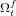$\Omega^f_t$ the domain occupied by the fluid and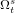$\Omega^s_t$ by the solid at the time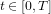$t\in[0,T]$. Let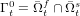$\Gamma^0_t=\bar{\Omega}^f_t \cap \bar{\Omega}^s_t$ be the part of the boundary where the elastic solid interacts with the fluid.

### Fluid properties

The fluid is considered to be Newtonian, incompressible and its state is described by the velocity and pressure fields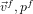$\vec{v}^f, p^f$. The balance equations are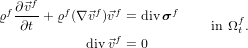$\begin{eqnarray*} \begin{split} \label{p0:f:blm0} \varrho^f \pp{\vec{v}^f}{t}+ \varrho^f ( \grad \vec{v}^f) \vec{v}^f &= \div \tens{\sigma}^f \\ \div \vec{v}^f&= 0 \end{split} \qquad \text{ in } \Omega^f_t . \end{eqnarray*}$

The material constitutive equation is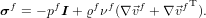\begin{align*} \tens{\sigma}^f=- p^f \tens{I} + \varrho^f \nu^f ( \grad \vec{v}^f + \trans{\grad \vec{v}^f} ). \end{align*}

The constant density of the fluid is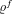$\varrho^f$ and the viscosity is denoted by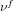$\nu^f$. The Reynolds number is defined by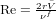$\Re = \frac{2 r \bar{V}}{\nu^f}$, with the mean velocity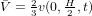$\bar{V}=\frac23 v(0,\frac{H}{2},t)$,$r$ radius of the cylinder and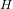$H$ height of the channel (see Figure 1).

### Structure properties

The structure is assumed to be elastic and compressible. Its configuration is described by the displacement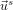$\vec{u}^s$, with velocity field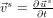$\vec{v}^s=\pp{\vec{u}^s}{t}$. The balance equations are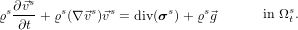$\begin{eqnarray*} \begin{split} \varrho^s \pp{\vec{v}^s}{t}+ \varrho^s ( \grad \vec{v}^s) \vec{v}^s&= \div (\tens{\sigma}^s) + \varrho^s \vec{g} %\\ \div \vec{v}^s&= 0 \end{split} \qquad \text{ in } \Omega^s_t . \end{eqnarray*}$

Written in the more common Lagrangian description, i.e. with respect to some fixed reference (initial) state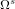$\Omega^s$, we have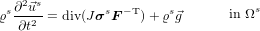$\begin{eqnarray*} \begin{split} \varrho^s \p2p{\vec{u}^s}{t} &= \div ( J \tens{\sigma}^s \transm{\tens{F}}) + \varrho^s \vec{g} %%\\ \det \tens{F}&= 1 \end{split} \qquad \text{ in } \Omega^s \end{eqnarray*}$
where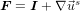$\tens{F}=\tens{I}+\nabla \vec{u}^s$ is the deformation gradient tensor. For further details see for example [Ciarlet88].

The material is specified by giving the Cauchy stress tensor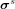$\tens{\sigma}^s$ (the 2nd Piola-Kirchhoff stress tensor is then given by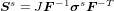$\tens{S}^s=J\tens{F}^{-1}\tens{\sigma}^s\tens{F}^{-T}$) by the following constitutive law for the St. Venant-Kirchhoff material (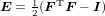$\tens{E}=\frac12 ( \trans{\tens{F} }\tens{F} - \tens{I})$)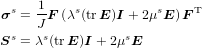\begin{align*} \tens{\sigma}^s &= \frac{1}{J} \tens{F} \left( \lambda^s ( \tr \tens{E} ) \tens{I} + 2 \mu^s \tens{E} \right) \trans{\tens{F}} \\ \tens{S}^s &= \lambda^s ( \tr \tens{E} ) \tens{I} + 2 \mu^s \tens{E} \end{align*}

The density of the structure in the undeformed configuration is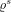$\varrho^s$. The elasticity of the material is characterized by the Poisson ratio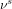$\nu^s$ (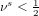$\nu^s<\frac12$ for a compressible structure) and by the Young modulus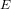$E$. The alternative characterization is described by the Lam� coefficients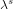$\lambda^s$ and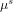$\mu^s$ (the shear modulus):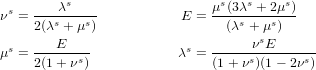\begin{align*} \nu^s&=\frac{\lambda^s}{2(\lambda^s+\mu^s)} & E&=\frac{\mu^s(3\lambda^s+2\mu^s)}{(\lambda^s+\mu^s)}\\ \mu^s&=\frac{E}{2(1+\nu^s)} & \lambda^s&=\frac{\nu^s E}{(1+\nu^s)(1-2\nu^s)} \end{align*}

### Interaction conditions

The boundary conditions on the fluid solid interface are assumed to be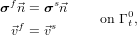$\begin{eqnarray*} \begin{split} \tens{\sigma}^f \vec{n} &= \tens{\sigma}^s \vec{n} \\ \vec{v}^f&= \vec{v}^s \end{split} \qquad \text{ on } \Gamma^0_t, \end{eqnarray*}$
where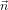$\vec{n}$ is a unit normal vector to the interface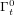$\Gamma^0_t$. This implies the no-slip condition for the flow, and that the forces on the interface are in balance.

### Domain definition

The domain is based on the 2D version of the well-known CFD benchmark in [TurekSchaefer1996] and shown here in Figure 1. By omitting the elastic bar behind the cylinder one can exactly recover the setup of the flow around cylinder configuration which allows for validation of the flow part by comparing the results with the older flow benchmark.• The domain dimensions are: length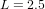$L=2.5$, height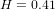$H=0.41$.
• The circle center is positioned at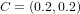$C=(0.2,0.2)$ (measured from the left bottom corner of the channel) and the radius is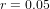$r=0.05$.
• The elastic structure bar has length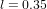$l=0.35$ and height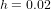$h=0.02$ , the right bottom corner is positioned at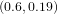$(0.6,0.19)$ , and the left end is fully attached to the fixed cylinder.
• The control points are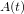$A(t)$, fixed with the structure with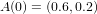$A(0)=(0.6,0.2)$ , and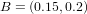$B=(0.15,0.2)$ .

The setting is intentionally non-symmetric (see [TurekSchaefer1996]) to prevent the dependence of the onset of any possible oscillation on the precision of the computation.

geometry parametersvalue [m]
channel lengthL2.5
channel widthH0.41
cylinder center positionC(0.2,0.2)
elastic structure lengthl0.35
elastic structure thicknessh0.02
reference point (at t=0)A(0.6,0.2)
reference pointB(0.2,0.2)

### Boundary conditions

• A parabolic velocity profile is prescribed at the left channel inflow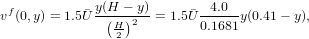\begin{align*} v^f(0,y)=1.5 \bar{U} \frac{y (H-y)}{\left(\frac{H}{2}\right)^2} =1.5 \bar{U} \frac{4.0}{0.1681} y (0.41-y), \label{vp} \end{align*}
such that the mean inflow velocity is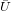$\bar{U}$ and the maximum of the inflow velocity profile is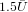$1.5\bar{U}$.
• The outflow condition can be chosen by the user, for example stress free or do nothing conditions. The outflow condition effectively prescribes some reference value for the pressure variable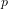$p$. While this value could be arbitrarily set in the incompressible case, in the case of compressible structure this will have influence on the stress and consequently the deformation of the solid. In this proposal, we set the reference pressure at the outflow to have zero mean value.
• The no-slip condition is prescribed for the fluid on the other boundary parts. i.e. top and bottom wall, circle and fluid-structure interface$\Gamma_t^0$.

### Initial conditions

Suggested starting procedure for the non-steady tests is to use a smooth increase of the velocity profile in time as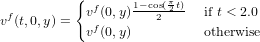\begin{align*} v^f(t,0,y) = \begin{cases} v^f(0,y) \frac{1-\cos ( \frac{\pi}{2} t )}{2} & \text{ if } t<2.0 \\ v^f(0,y) & \text{ otherwise } \end{cases} \end{align*}
where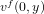$v^f(0,y)$ is the velocity profile.

### Material parameters

material$\varrho^s$ [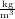$\frac{\textrm{kg}\nop}{\textrm{m}^3}$]$\nu^s$ E [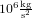$10^6 \frac{\textrm{kg}\nop}{\textrm{s}^2}$]$\mu^s$ [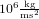$10^6 \frac{\textrm{kg}\nop}{\textrm{m}\textrm{s}^2}$]
polyurethane 1200 0.50 25 8.3
polypropylene 1100 0.42 900 317
PVC 1400 0.42 1500 528
steel 7800 0.29 210000 81400
cork 180 0.25 32 12.8

material$\varrho^s$ [$\frac{\textrm{kg}\nop}{\textrm{m}^3}$]$\nu^s$ E [$10^6 \frac{\textrm{kg}\nop}{\textrm{s}^2}$]$\mu^s$ [$10^6 \frac{\textrm{kg}\nop}{\textrm{m}\textrm{s}^2}$]
air 1.23 0.015 0.018
aceton 790 0.405 0.32
ethyl alcohol 790 1.4 1.1
oil, vegetable 920 76.170
water 1000 1.14 1.14
blood 1035 3 -- 4 3 -- 4
glycerine 1260 11271420
honey 1420 704210000
mercury 13594 0.0114 1.55

An overview of certain material properties for some relevant fluids and elastic materials is shown in the Table. The choice of the parameters for the benchmark is guided by several requirements:

First, we would like the flow to be in the laminar regime, which implies "small" Reynolds numbers. On the other hand, the flow should be capable of deforming the elastic structure. A typical fluid candidate for such experiments is glycerine.

In order not to introduce additional numerical complications connected with high aspect ratios in the geometry, the deformable structure has a certain thickness which requires that the stiffness of the material should be low enough to allow significant deformations. Certain rubber-like materials fit into such a setting, namely polybutadiene (for a future incompressible configuration) and polypropylene.

In the table the material parameters are presented for 2 combinations of glycerine and selected rubber-like material.$\varrho^s$ [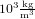$10^3 \frac{\textrm{kg}\nop}{\textrm{m}^3}$] 0.91 1.1$\nu^s$ 0.5 0.42$\mu^s$ [$10^6 \frac{\textrm{kg}\nop}{\textrm{ms}^2}$] 0.53 317$\varrho^f$ [$10^3 \frac{\textrm{kg}}{\textrm{m}^3}$] 1.26 1.26$\nu^f$ [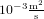$10^{-3} \frac{\textrm{m}^2}{\textrm{s}}$] 1.13 1.13